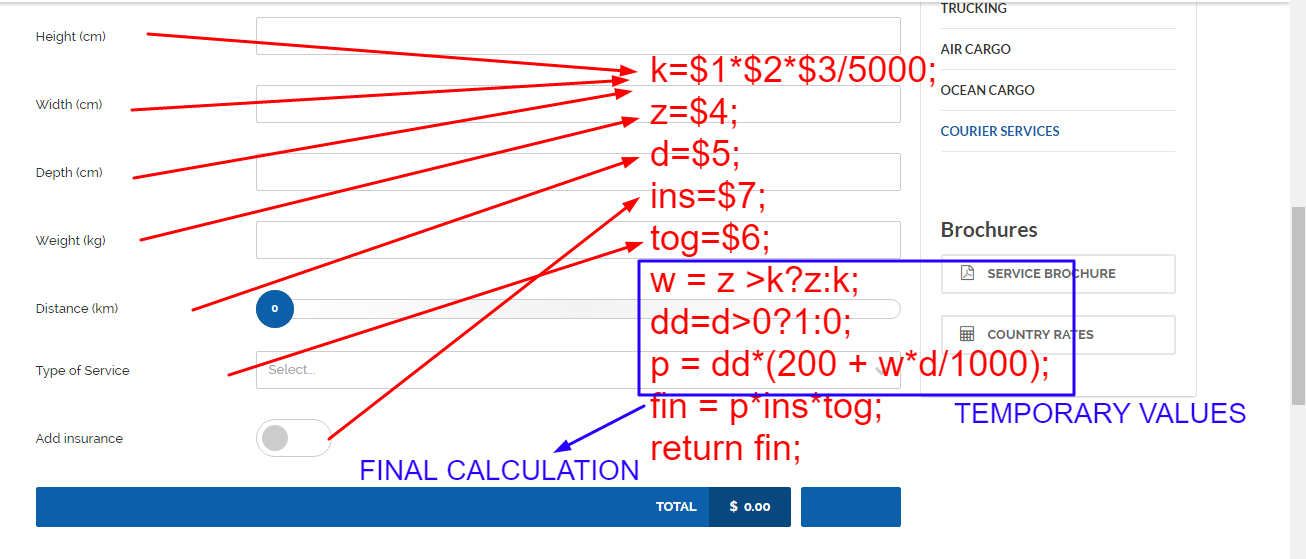Public Ticket #1068797
How to Use Calculator
Closed

•Hussein started the conversation

Hi,

I bought the Cargo theme, which comes built in with the Cost Calculator.  I have found out how to edit the names of the different variables, but i do not know how to change the formula for calculation.

I have searched online and found that there should be a panel for setting for the cost calculator, but i do not have it on my site.

Thanks

•Support Agent 1 replied

Hi,

We are sending you the .pdf theme documentation file which can help you to get to know Cost Calculator. Please note: the attached documentation is explained for users which are not using our theme, but Cost Calculator itselfs and Visual Composer as a page builder - but the process of building Cost Calculator is the same.

If you have installed demo content, here is the explanation about JS Pseudo code from Cost Calculator Group:

```k = \$1*\$2*\$3/5000;
z = \$4;
d = \$5;
ins = \$7;
tog = \$6;
w = z >k?z:k;
dd=d>0?1:0;
p = dd*(200 + w*d/1000);
final = p*ins*tog;
return final;
```

And here is the screeshot with explanation: